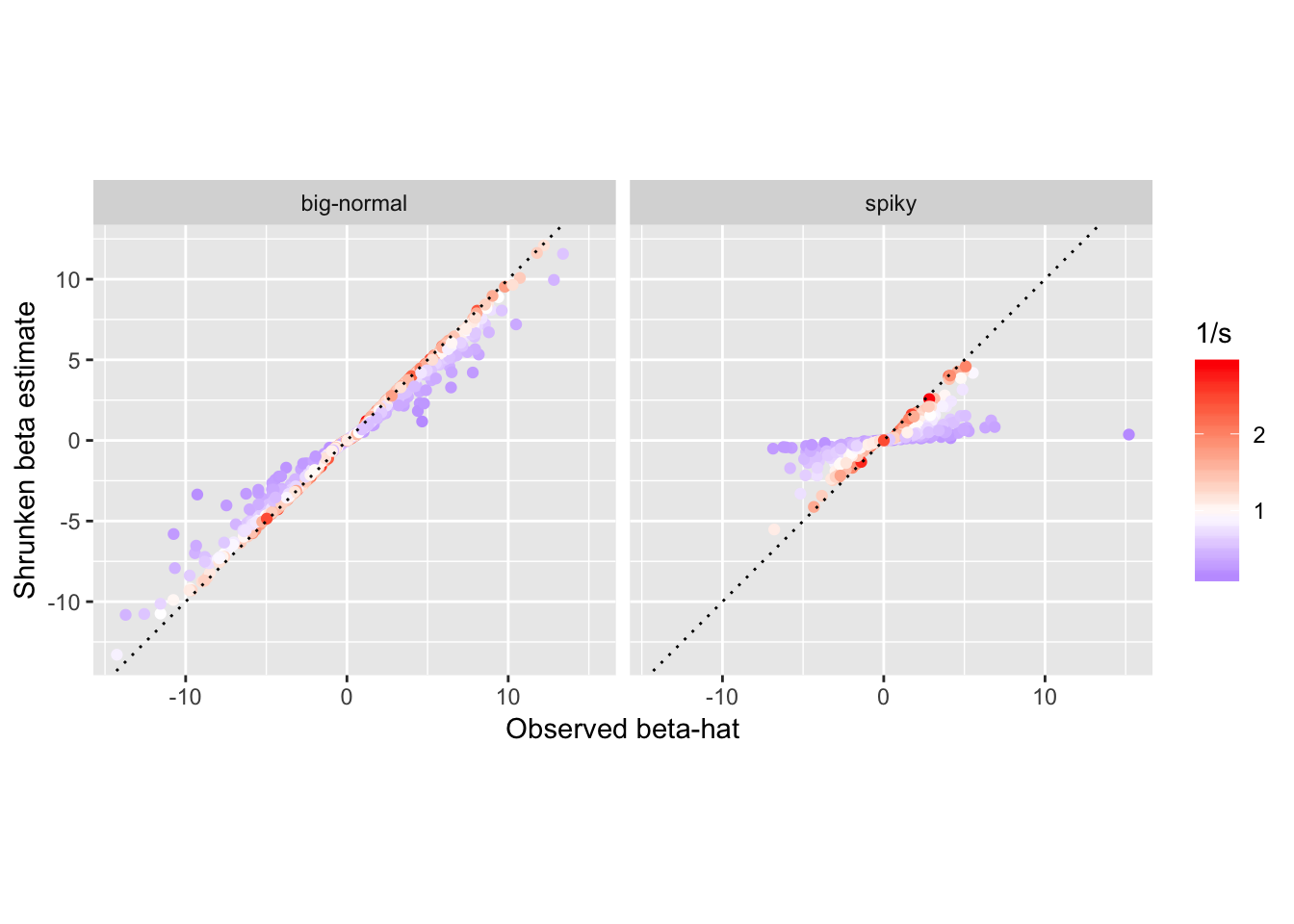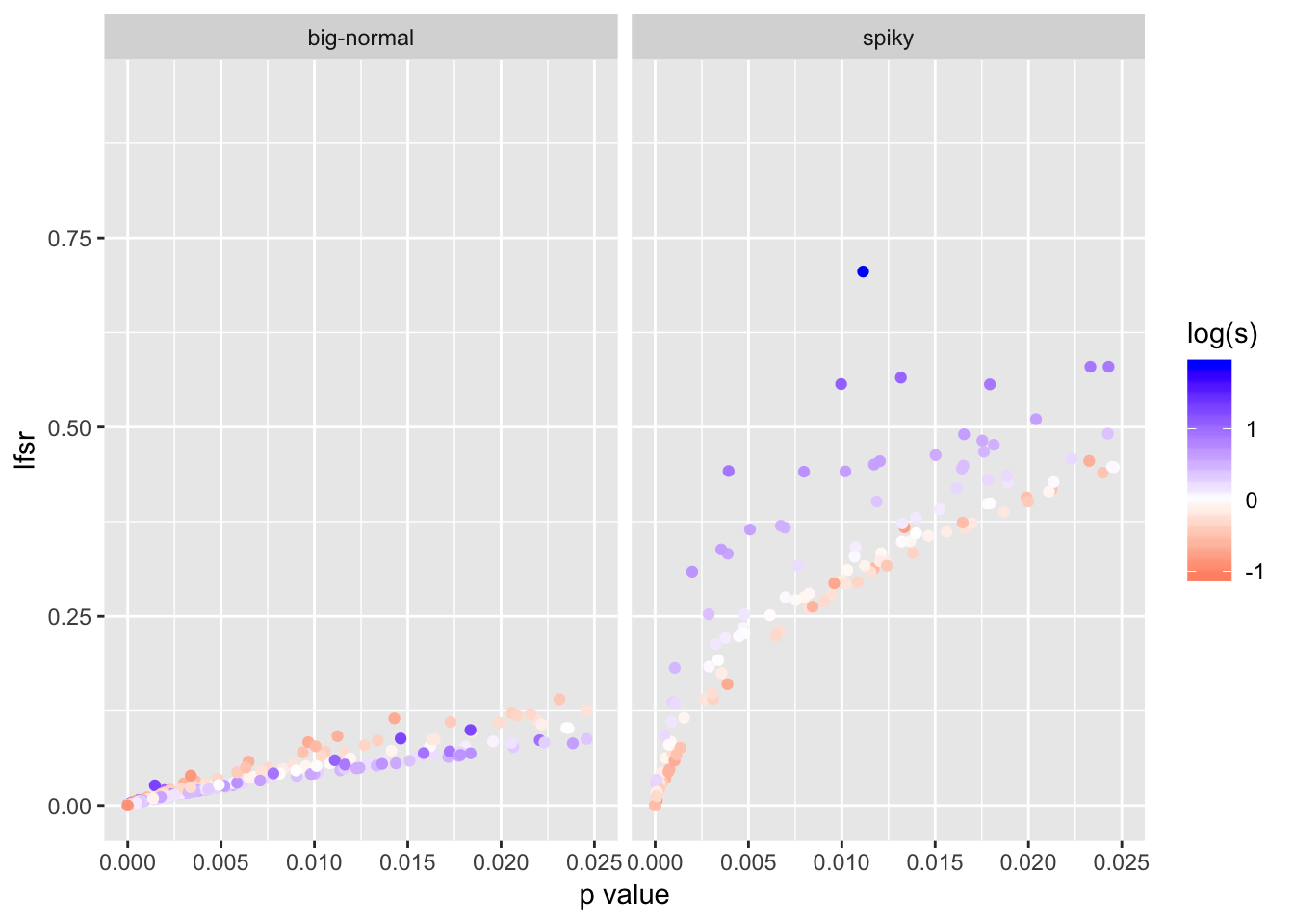The goal here is to illustrate the “adaptive” nature of the adaptive shrinkage. The shrinkage is adaptive in two senses. First, the amount of shrinkage depends on the distribution \(g\) of the true effects, which is learned from the data: when \(g\) is very peaked about zero then ash learns this and deduces that signals should be more strongly shrunk towards zero than when \(g\) is less peaked about zero. Second, the amount of shrinkage of each observation depends on its standard error: the smaller the standard error, the more informative the data, and so the less shrinkage that occurs. From an Empirical Bayesian perspective both of these points are entirely natural: the posterior depends on both the prior and the likelihood; the prior, \(g\), is learned from the data, and the likelihood incorporates the standard error of each observation.

First, we load the necessary libraries.

``````library(ashr)
library(ggplot2)``````
``## Warning: package 'ggplot2' was built under R version 3.4.4``

We simulate from two scenarios: in the first scenario, the effects are more peaked about zero (sim.spiky); in the second scenario, the effects are less peaked at zero (sim.bignormal). A summary of the two data sets is printed at the end of this chunk.

``````set.seed(100)

# Simulates data sets for experiments below.
rnormmix_datamaker = function (args) {

# generate the proportion of true nulls randomly.
pi0 = runif(1,args\$min_pi0,args\$max_pi0)
k   = ncomp(args\$g)

#randomly draw a component
comp   = sample(1:k,args\$nsamp,mixprop(args\$g),replace = TRUE)
isnull = (runif(args\$nsamp,0,1) < pi0)
beta   = ifelse(isnull,0,rnorm(args\$nsamp,comp_mean(args\$g)[comp],
comp_sd(args\$g)[comp]))
sebetahat = args\$betahatsd
betahat   = beta + rnorm(args\$nsamp,0,sebetahat)
meta      = list(g1 = args\$g,beta = beta,pi0 = pi0)
input     = list(betahat = betahat,sebetahat = sebetahat,df = NULL)
return(list(meta = meta,input = input))
}

NSAMP = 1000
s     = 1/rgamma(NSAMP,5,5)

sim.spiky =
rnormmix_datamaker(args = list(g = normalmix(c(0.4,0.2,0.2,0.2),
c(0,0,0,0),
c(0.25,0.5,1,2)),
min_pi0   = 0,
max_pi0   = 0,
nsamp     = NSAMP,
betahatsd = s))

sim.bignormal =
rnormmix_datamaker(args = list(g         = normalmix(1,0,4),
min_pi0   = 0,
max_pi0   = 0,
nsamp     = NSAMP,
betahatsd = s))

cat("Summary of observed beta-hats:\n")
## Summary of observed beta-hats:
print(rbind(spiky     = quantile(sim.spiky\$input\$betahat,seq(0,1,0.1)),
bignormal = quantile(sim.bignormal\$input\$betahat,seq(0,1,0.1))),
digits = 3)
##               0%   10%   20%    30%    40%     50%   60%   70%  80%  90%
## spiky      -6.86 -1.99 -1.19 -0.717 -0.326  0.0380 0.385 0.728 1.23 2.04
## bignormal -14.26 -5.03 -3.48 -1.989 -0.984 -0.0941 1.026 2.141 3.63 5.61
##           100%
## spiky     15.2
## bignormal 13.4``````

Now we run ash on both data sets.

``````beta.spiky.ash     = ash(sim.spiky\$input\$betahat,s)
beta.bignormal.ash = ash(sim.bignormal\$input\$betahat,s)``````

Next we plot the shrunken estimates against the observed values, colored according to the (square root of) precision: precise estimates being colored red, and less precise estimates being blue. Two key features of the plots illustrate the ideas of adaptive shrinkage: i) the estimates under the spiky scenario are shrunk more strongly, illustrating that shrinkage adapts to the underlying distribution of beta; ii) in both cases, estimates with large standard error (blue) are shrunk more than estimates with small standard error (red) illustrating that shrinkage adapts to measurement precision.

``````make_df_for_ashplot =
function (sim1, sim2, ash1, ash2, name1 = "spiky", name2 = "big-normal") {
n = length(sim1\$input\$betahat)
x = c(get_lfsr(ash1),get_lfsr(ash2))
return(data.frame(betahat  = c(sim1\$input\$betahat,sim2\$input\$betahat),
beta_est = c(get_pm(ash1),get_pm(ash2)),
lfsr     = x,
s        = c(sim1\$input\$sebetahat,sim2\$input\$sebetahat),
scenario = c(rep(name1,n),rep(name2,n)),
signif   = x < 0.05))
}

ashplot = function(df,xlab="Observed beta-hat",ylab="Shrunken beta estimate")
ggplot(df,aes(x = betahat,y = beta_est,color = 1/s)) +
xlab(xlab) + ylab(ylab) + geom_point() +
facet_grid(.~scenario) +
geom_abline(intercept = 0,slope = 1,linetype = "dotted") +
mid = "white",high = "red",space = "Lab") +
coord_fixed(ratio = 1)

df = make_df_for_ashplot(sim.spiky,sim.bignormal,beta.spiky.ash,
beta.bignormal.ash)
print(ashplot(df))``````A related consequence is that significance of each observation is no longer monotonic with \(p\) value.

``````pval_plot = function (df)
ggplot(df,aes(x = pnorm(-abs(betahat/s)),y = lfsr,color = log(s))) +
geom_point() + facet_grid(.~scenario) + xlim(c(0,0.025)) +
xlab("p value") + ylab("lfsr") +
mid = "white",high = "blue")

print(pval_plot(df))``````Let’s see how these are affected by changing the modelling assumptions so that the standardized beta are exchangeable (rather than the beta being exchangeable).

``````beta.bignormal.ash.ET =
ash(sim.bignormal\$input\$betahat,s,alpha = 1,mixcompdist = "normal")
beta.spiky.ash.ET =
ash(sim.spiky\$input\$betahat,s,alpha = 1,mixcompdist = "normal")
df.ET = make_df_for_ashplot(sim.spiky,sim.bignormal,beta.spiky.ash.ET,
beta.bignormal.ash.ET)
ashplot(df.ET,ylab = "Shrunken beta estimate (ET model)")``````